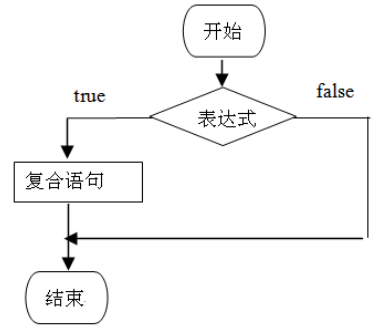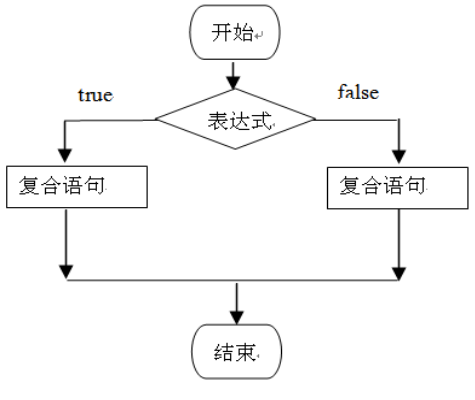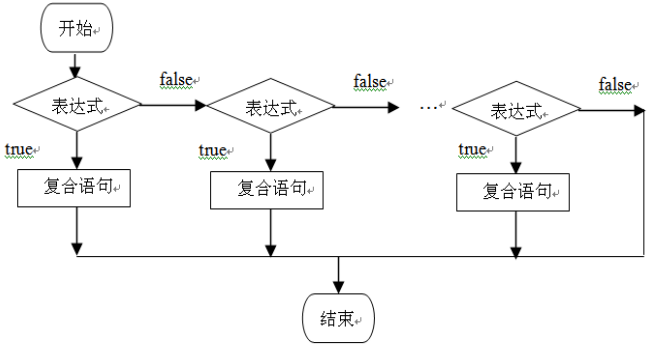# 选择结构

## if条件分支语句

• if语句
• if-else语句
• if-else if- ……- else if -else 语句

### if语句

if语句是单条件分支语句，即根据一个条件来控制程序执行的流程if 语句的语法格式：

``````    if（表达式）{
若干语句
}
``````

``````//将变量a，b，c内存中的数值按大小顺序进行互换（从小到大排列）。
public class Example3_2 {
public static void main(String args[]) {
int a = 9,b = 5,c = 7,t=0;
if(b<a) {
t = a;  a = b; b = t;
}
if(c<a) {
t = a; a = c;c = t;
}
if(c<b) {
t = b;  b = c; c = t;
}
System.out.println("a="+a+",b="+b+",c="+c);
}
}

``````

### if-else 语句

if-else 语句是双条件分支语句，即根据一个条件来控制程序执行的流程。if-else 语句的语法格式：

``````  if（表达式） {
若干语句
}
else {
若干语句
}

``````

### if-else if-else 语句

if-elseif 语句是多条件分支语句，即根据多个条件来控制程序执行的流程。if-else if-else语句的语法格式：

``````if（表达式) {
若干语句
}else if（表达式) {
若干语句
}
… …
else {
若干语句
}

``````

## switch开关语句

switch 语句是单条件多分支的开关语句

``````switch(表达式){
case 常量值1:
若干个语句
break;
case  常量值2:
若干个语句
break;
...
case  常量值n:
若干个语句
break;
default:
若干语句
}

``````

2)没有break语句，就会匹配显示从匹配的常量值到最后。

3)default语句放的位置是任意的。

4)检查时case 常量必须与switch表达式可用的类型相同。

5)根据2)和4)，switch的结束只有两种情况，到语句末尾，碰到break.

``````import java.util.Scanner;

public class test {
public static void main(String args[]) {
int number = 0;
switch (number) {
case 1:
System.out.println("1");
break;
case 2:
System.out.println("2");
break;
default:
System.out.println("666");
//如果不是123，不会执行完本句就结束，而是一直到末尾或者碰到break
case 3:
System.out.println("3");
break;
}
}
}
/*

6

666
3
*/
``````